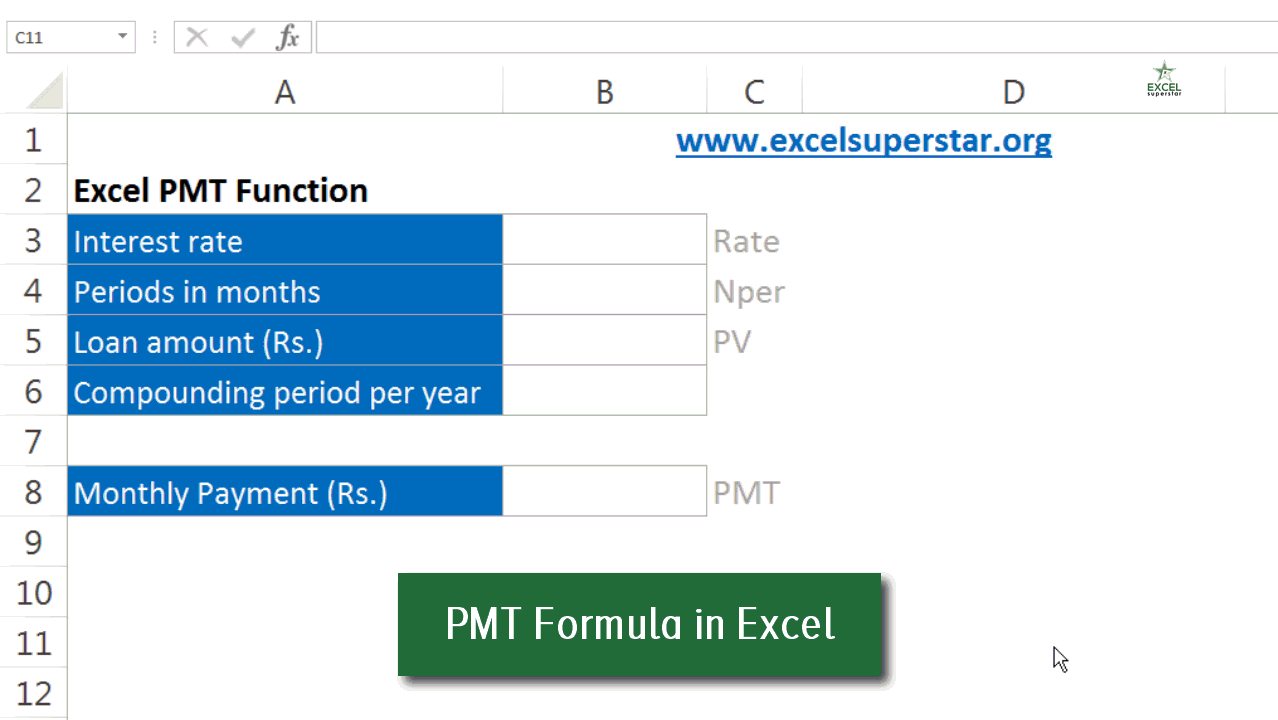# How to use PMT Function in ExcelPMT Function denotes the annuity payment that you need to make including the interest during a particular period of time. This is a very common function in Excel. If you know the interest rate, a number of years, and the present value you can easily calculate the Annuity payment you need to make.

Where,50% OFF on Excel Course

• Get 2-Year Access with unlimited views of 220+ videos
• Use Coupon code - NAV50OFF & Start learning

PMT stands for Annuity Payment.

Rate stands for the interest rate for the period.

Nper stands for the number of years or the time period for the loan.

PV stands for the Present Value.

FV is an optional argument which stands for future value.

Type is also an optional argument which stands for when the payment is made at the end, in the beginning. It is assumed that the payment is made at the end of the period.Example of PMT Function

A loan of Rs. 2,00,000 with monthly payment was taken at an annual interest rate of 9% for a duration of 15 years. What would be the PMT?

Solution:

Here, the Rate =0.75% as we need to make monthly payment so 9%/12 will be 0.75%

Nper = 180 as we need to make monthly payment so 15*12 will be 180

PV = Rs. 2,00,000

The other two arguments in the box bracket are optional.